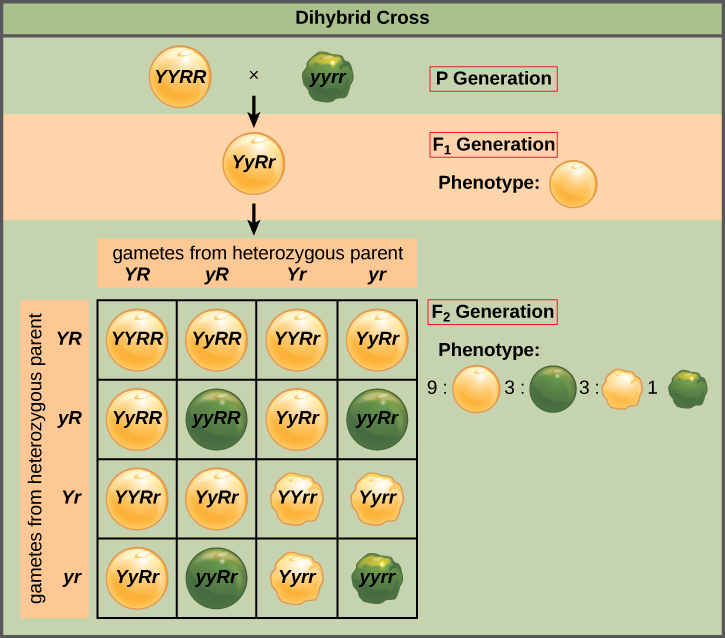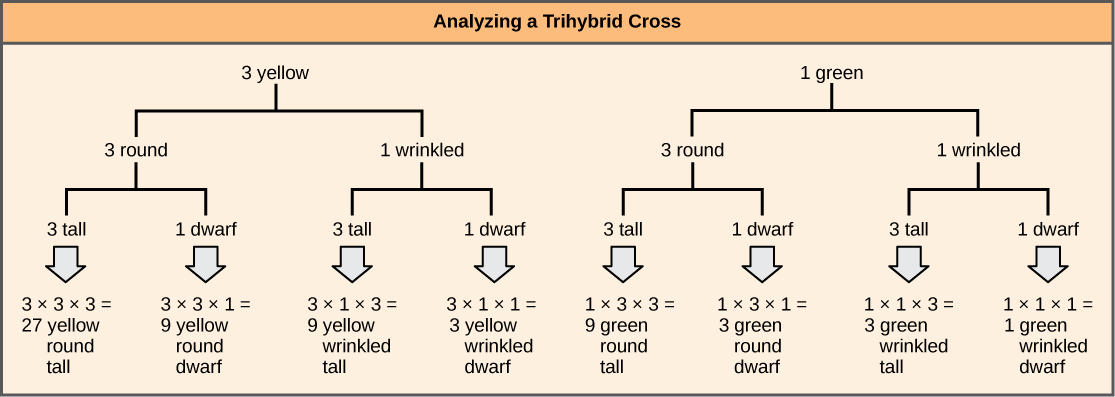# Independent Assortment

Mendel’s law of independent assortment states that genes do not influence each other with regard to the sorting of alleles into gametes, and every possible combination of alleles for every gene is equally likely to occur. The independent assortment of genes can be illustrated by the dihybrid cross, a cross between two true-breeding parents that express different traits for two characteristics. Consider the characteristics of seed color and seed texture for two pea plants, one that has green, wrinkled seeds (yyrr) and another that has yellow, round seeds (YYRR). Because each parent is homozygous, the law of segregation indicates that the gametes for the green/wrinkled plant all are yr, and the gametes for the yellow/round plant are all YR. Therefore, the F1 generation of offspring all are YyRr (Figure).

## Art ConnectionThis dihybrid cross of pea plants involves the genes for seed color and texture.

In pea plants, purple flowers (P) are dominant to white flowers (p) and yellow peas (Y) are dominant to green peas (y). What are the possible genotypes and phenotypes for a cross between PpYY and ppYy pea plants? How many squares do you need to do a Punnett square analysis of this cross?

For the F2 generation, the law of segregation requires that each gamete receive either an R allele or an r allele along with either a Y allele or a y allele. The law of independent assortment states that a gamete into which an r allele sorted would be equally likely to contain either a Y allele or a y allele. Thus, there are four equally likely gametes that can be formed when the YyRr heterozygote is self-crossed, as follows: YR, Yr, yR, and yr. Arranging these gametes along the top and left of a 4 × 4 Punnett square (Figure) gives us 16 equally likely genotypic combinations. From these genotypes, we infer a phenotypic ratio of 9 round/yellow:3 round/green:3 wrinkled/yellow:1 wrinkled/green (Figure). These are the offspring ratios we would expect, assuming we performed the crosses with a large enough sample size.

Because of independent assortment and dominance, the 9:3:3:1 dihybrid phenotypic ratio can be collapsed into two 3:1 ratios, characteristic of any monohybrid cross that follows a dominant and recessive pattern. Ignoring seed color and considering only seed texture in the above dihybrid cross, we would expect that three quarters of the F2 generation offspring would be round, and one quarter would be wrinkled. Similarly, isolating only seed color, we would assume that three quarters of the F2 offspring would be yellow and one quarter would be green. The sorting of alleles for texture and color are independent events, so we can apply the product rule. Therefore, the proportion of round and yellow F2 offspring is expected to be (3/4) × (3/4) = 9/16, and the proportion of wrinkled and green offspring is expected to be (1/4) × (1/4) = 1/16. These proportions are identical to those obtained using a Punnett square. Round, green and wrinkled, yellow offspring can also be calculated using the product rule, as each of these genotypes includes one dominant and one recessive phenotype. Therefore, the proportion of each is calculated as (3/4) × (1/4) = 3/16.

The law of independent assortment also indicates that a cross between yellow, wrinkled (YYrr) and green, round (yyRR) parents would yield the same F1 and F2 offspring as in the YYRR x yyrr cross.

The physical basis for the law of independent assortment also lies in meiosis I, in which the different homologous pairs line up in random orientations. Each gamete can contain any combination of paternal and maternal chromosomes (and therefore the genes on them) because the orientation of tetrads on the metaphase plane is random.

## Forked-Line Method

When more than two genes are being considered, the Punnett-square method becomes unwieldy. For instance, examining a cross involving four genes would require a 16 × 16 grid containing 256 boxes. It would be extremely cumbersome to manually enter each genotype. For more complex crosses, the forked-line and probability methods are preferred.

To prepare a forked-line diagram for a cross between F1 heterozygotes resulting from a cross between AABBCC and aabbcc parents, we first create rows equal to the number of genes being considered, and then segregate the alleles in each row on forked lines according to the probabilities for individual monohybrid crosses (Figure). We then multiply the values along each forked path to obtain the F2 offspring probabilities. Note that this process is a diagrammatic version of the product rule. The values along each forked pathway can be multiplied because each gene assorts independently. For a trihybrid cross, the F2 phenotypic ratio is 27:9:9:9:3:3:3:1.The forked-line method can be used to analyze a trihybrid cross. Here, the probability for color in the F2 generation occupies the top row (3 yellow:1 green). The probability for shape occupies the second row (3 round:1 wrinked), and the probability for height occupies the third row (3 tall:1 dwarf). The probability for each possible combination of traits is calculated by multiplying the probability for each individual trait. Thus, the probability of F2 offspring having yellow, round, and tall traits is 3 × 3 × 3, or 27.

## Probability Method

While the forked-line method is a diagrammatic approach to keeping track of probabilities in a cross, the probability method gives the proportions of offspring expected to exhibit each phenotype (or genotype) without the added visual assistance. Both methods make use of the product rule and consider the alleles for each gene separately. Earlier, we examined the phenotypic proportions for a trihybrid cross using the forked-line method; now we will use the probability method to examine the genotypic proportions for a cross with even more genes.

For a trihybrid cross, writing out the forked-line method is tedious, albeit not as tedious as using the Punnett-square method. To fully demonstrate the power of the probability method, however, we can consider specific genetic calculations. For instance, for a tetrahybrid cross between individuals that are heterozygotes for all four genes, and in which all four genes are sorting independently and in a dominant and recessive pattern, what proportion of the offspring will be expected to be homozygous recessive for all four alleles? Rather than writing out every possible genotype, we can use the probability method. We know that for each gene, the fraction of homozygous recessive offspring will be 1/4. Therefore, multiplying this fraction for each of the four genes, (1/4) × (1/4) × (1/4) × (1/4), we determine that 1/256 of the offspring will be quadruply homozygous recessive.

For the same tetrahybrid cross, what is the expected proportion of offspring that have the dominant phenotype at all four loci? We can answer this question using phenotypic proportions, but let’s do it the hard way—using genotypic proportions. The question asks for the proportion of offspring that are 1) homozygous dominant at A or heterozygous at A, and 2) homozygous at B or heterozygous at B, and so on. Noting the “or” and “and” in each circumstance makes clear where to apply the sum and product rules. The probability of a homozygous dominant at A is 1/4 and the probability of a heterozygote at A is 1/2. The probability of the homozygote or the heterozygote is 1/4 + 1/2 = 3/4 using the sum rule. The same probability can be obtained in the same way for each of the other genes, so that the probability of a dominant phenotype at A and B and C and D is, using the product rule, equal to 3/4 × 3/4 × 3/4 × 3/4, or 27/64. If you are ever unsure about how to combine probabilities, returning to the forked-line method should make it clear.

## Rules for Multihybrid Fertilization

Predicting the genotypes and phenotypes of offspring from given crosses is the best way to test your knowledge of Mendelian genetics. Given a multihybrid cross that obeys independent assortment and follows a dominant and recessive pattern, several generalized rules exist; you can use these rules to check your results as you work through genetics calculations (Table). To apply these rules, first you must determine n, the number of heterozygous gene pairs (the number of genes segregating two alleles each). For example, a cross between AaBb and AaBb heterozygotes has an n of 2. In contrast, a cross between AABb and AABb has an n of 1 because A is not heterozygous.

General Rules for Multihybrid Crosses
General Rule Number of Heterozygous Gene Pairs
Number of different F1 gametes 2n
Number of different F2 genotypes 3n
Given dominant and recessive inheritance, the number of different F2 phenotypes 2n
5 of 11